title: “DS624_HW6_JagdishChhabria” author: “Jagdish Chhabria” date: “10/14/2021” output: pdf_document: default html_document: null toc_float: yes toc_collapsed: yes toc: yes toc_depth: 3 number_sections: yes theme: lumen

9.1) Figure 9.32 shows the ACFs for 36 random numbers, 360 random numbers and 1,000 random numbers.

a. Explain the differences among these figures. Do they all indicate that the data are white noise?

Given that the numbers used to represent this time series are random, the ACF plot expectedly shows no autocorrelation between them. The autocorrelation bars do not display any recurring pattern or seasonality, and none of them exceed the 95% confidence interval represented by the two blue horizontal lines. So all 3 plots indicate that the data is white noise.

b. Why are the critical values at different distances from the mean of zero? Why are the autocorrelations different in each figure when they each refer to white noise?

The critical values are at different distances from the mean of zero because they are based on the standard deviation of the autocorrelation coefficient at lag k, which in turn is inversely proportional to the number of observations in the time series. As N increases, the variance decreases and the bands get narrower. The autocorrelations are different in each figure possibly because the same random seed may not have been used in generating the 3 sets of random numbers. so while they each refer to white noise the actual value for the ACF at different lags is not the same across the 3 time series.

9.2) A classic example of a non-stationary series are stock prices. Plot the daily closing prices for Amazon stock (contained in gafa_stock), along with the ACF and PACF. Explain how each plot shows that the series is non-stationary and should be differenced.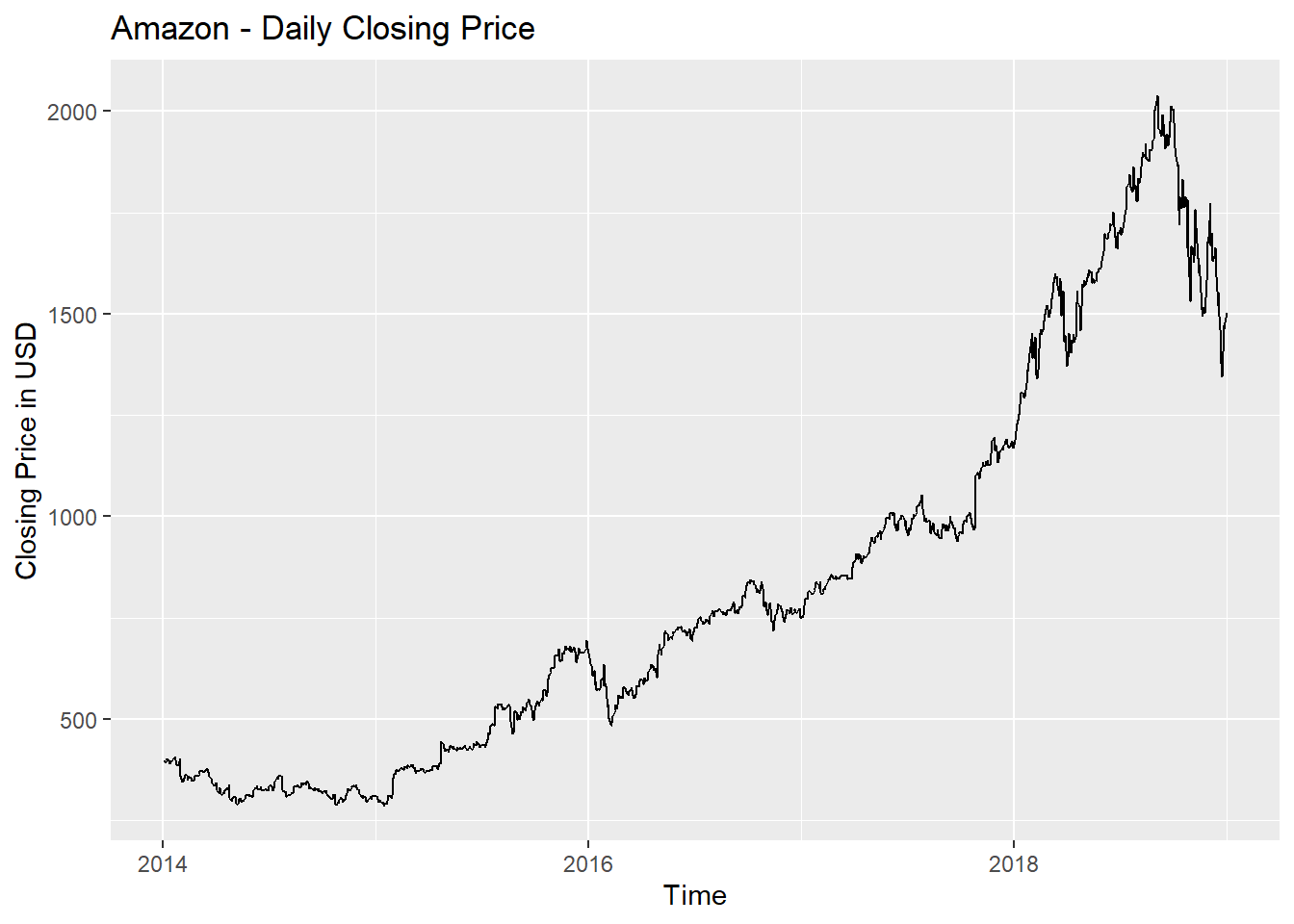The above plot shows a clear trend in the data - an upward trend from 2015 to mid-2018, and a downward trend from mid-2018 to 2019.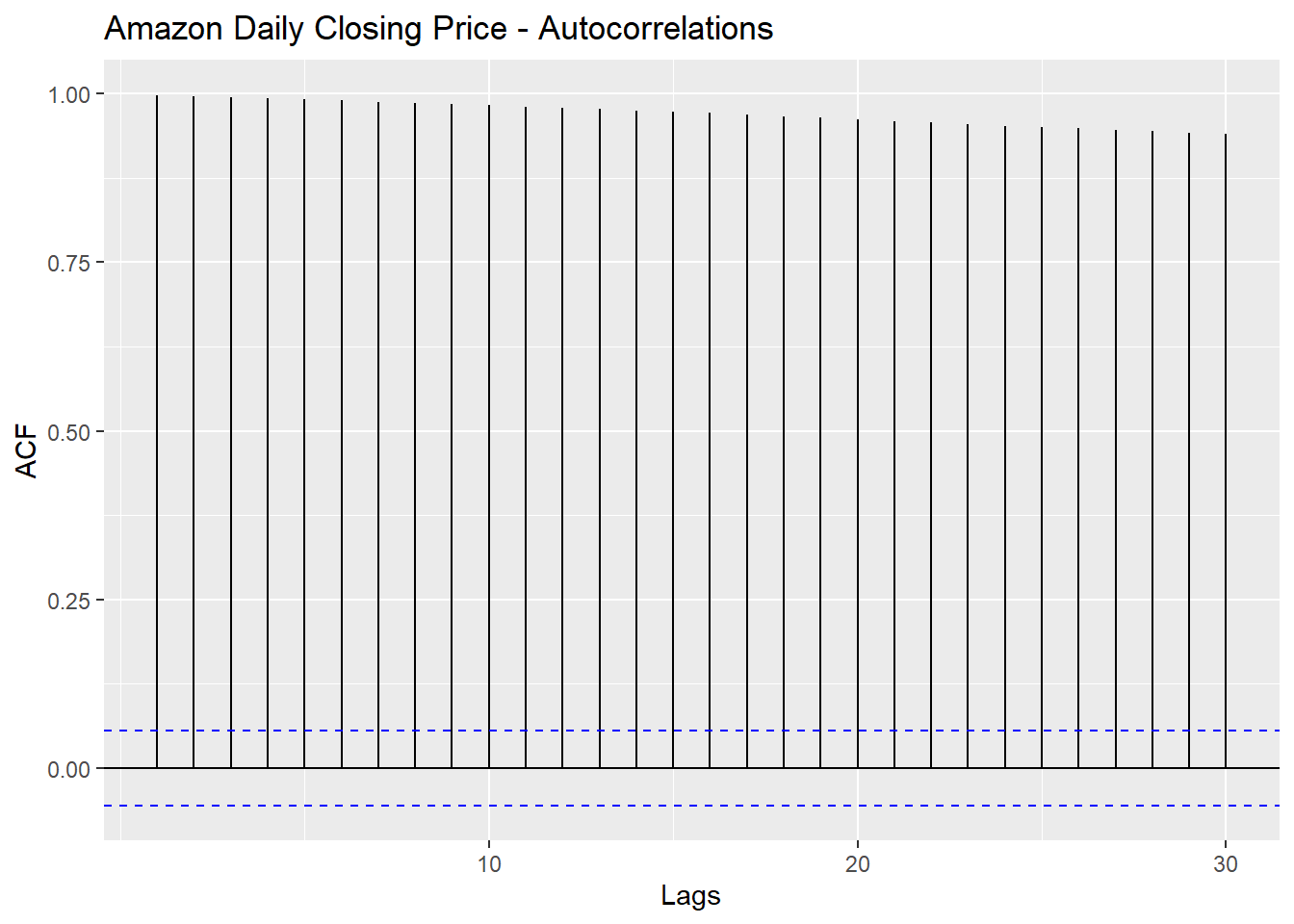The ACF plot shows significant autocorrelations for all lags going upto lag 30. This indicates that the data is not stationary.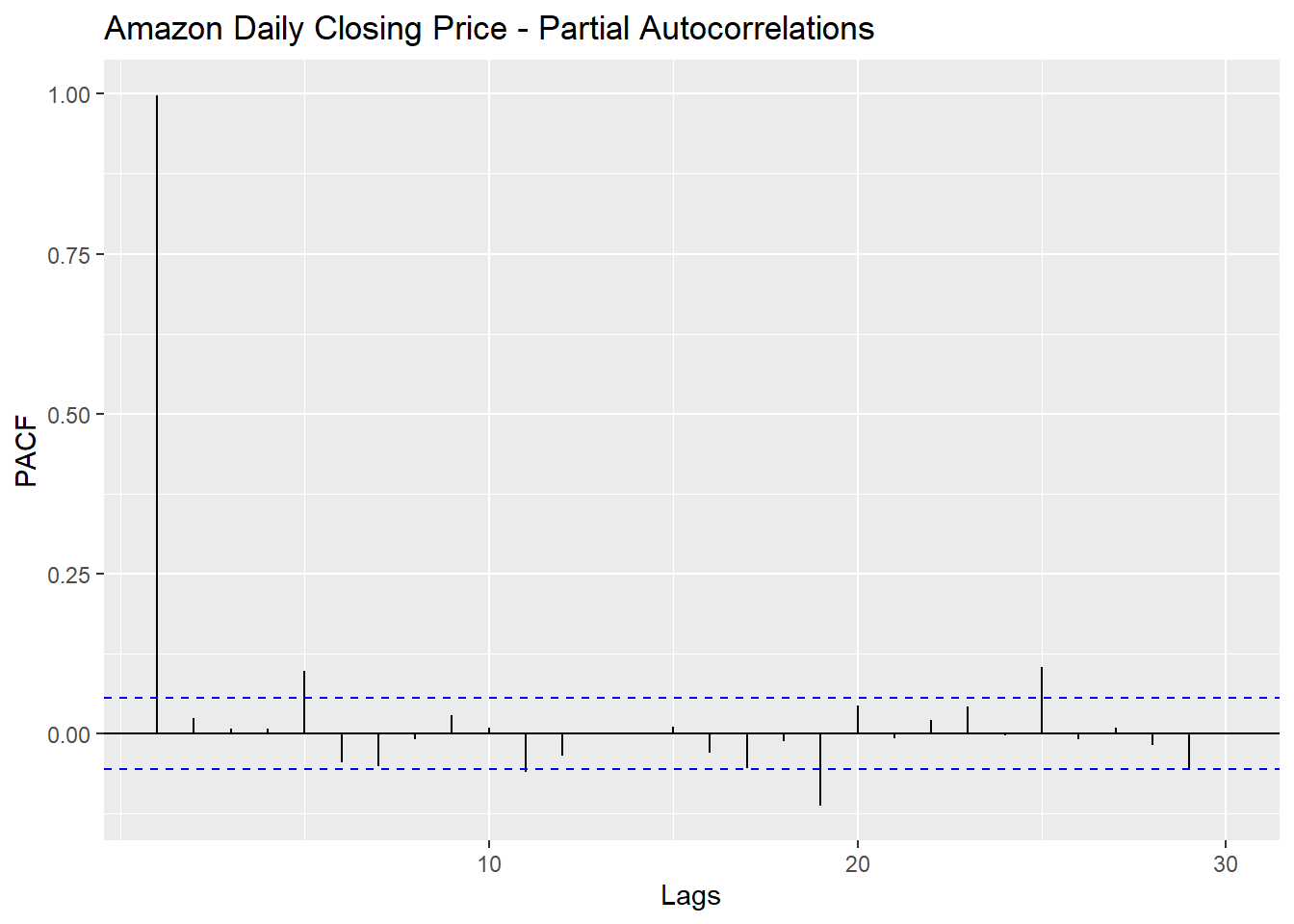The PACF plot shows significant partial autocorrelation for lag = 1 and then significant partial autocorrelations for lag = 5, 19 and 25.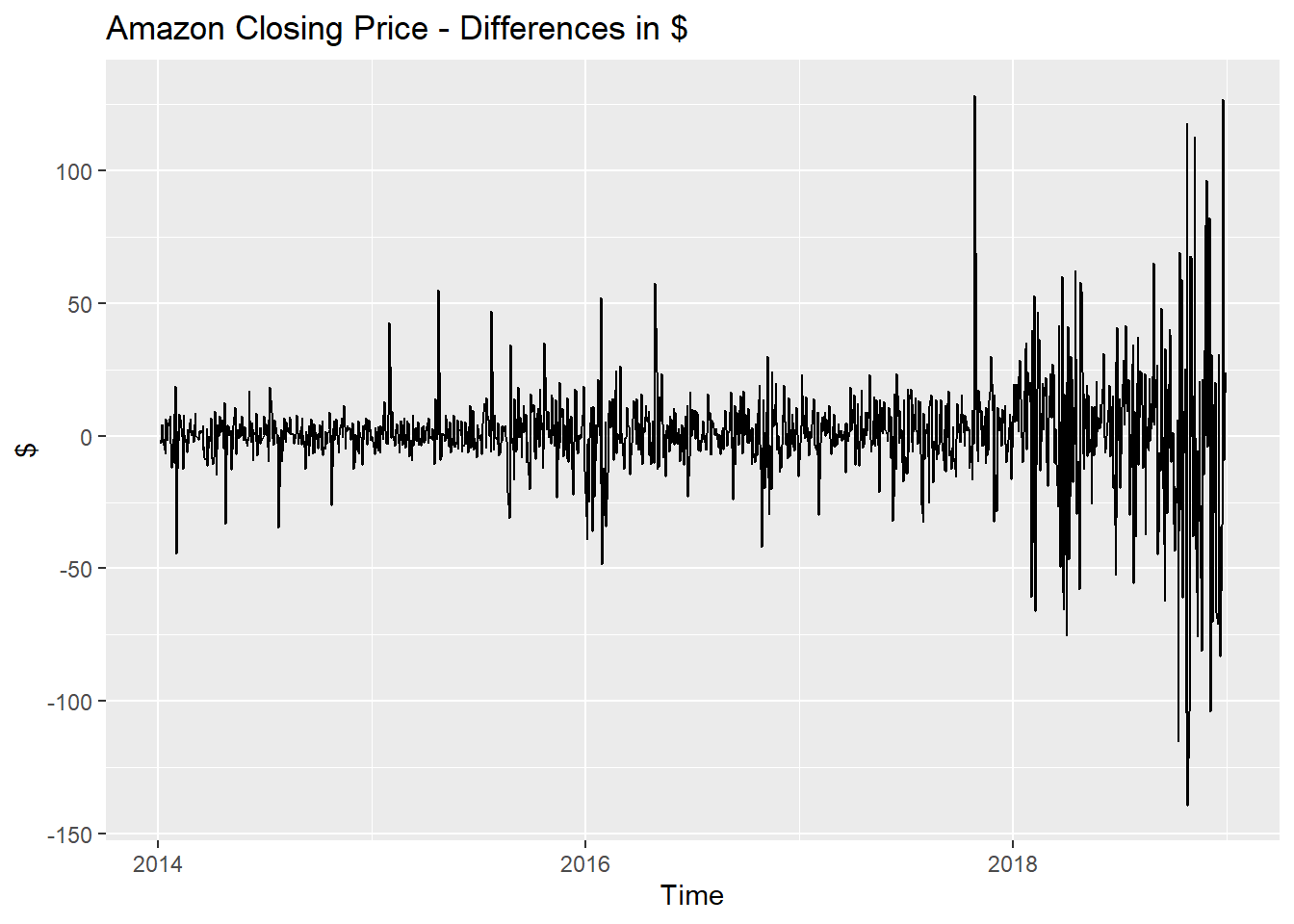We calculate how many rounds of differencing will need to be applied in order to achieve stationarity.

## # A tibble: 1 x 2
##   Symbol ndiffs
##   <chr>   <int>
## 1 AMZN        1

From the above, it seems just first-differencing should suffice. We re-plot the ACF and PACF after applying first differencing.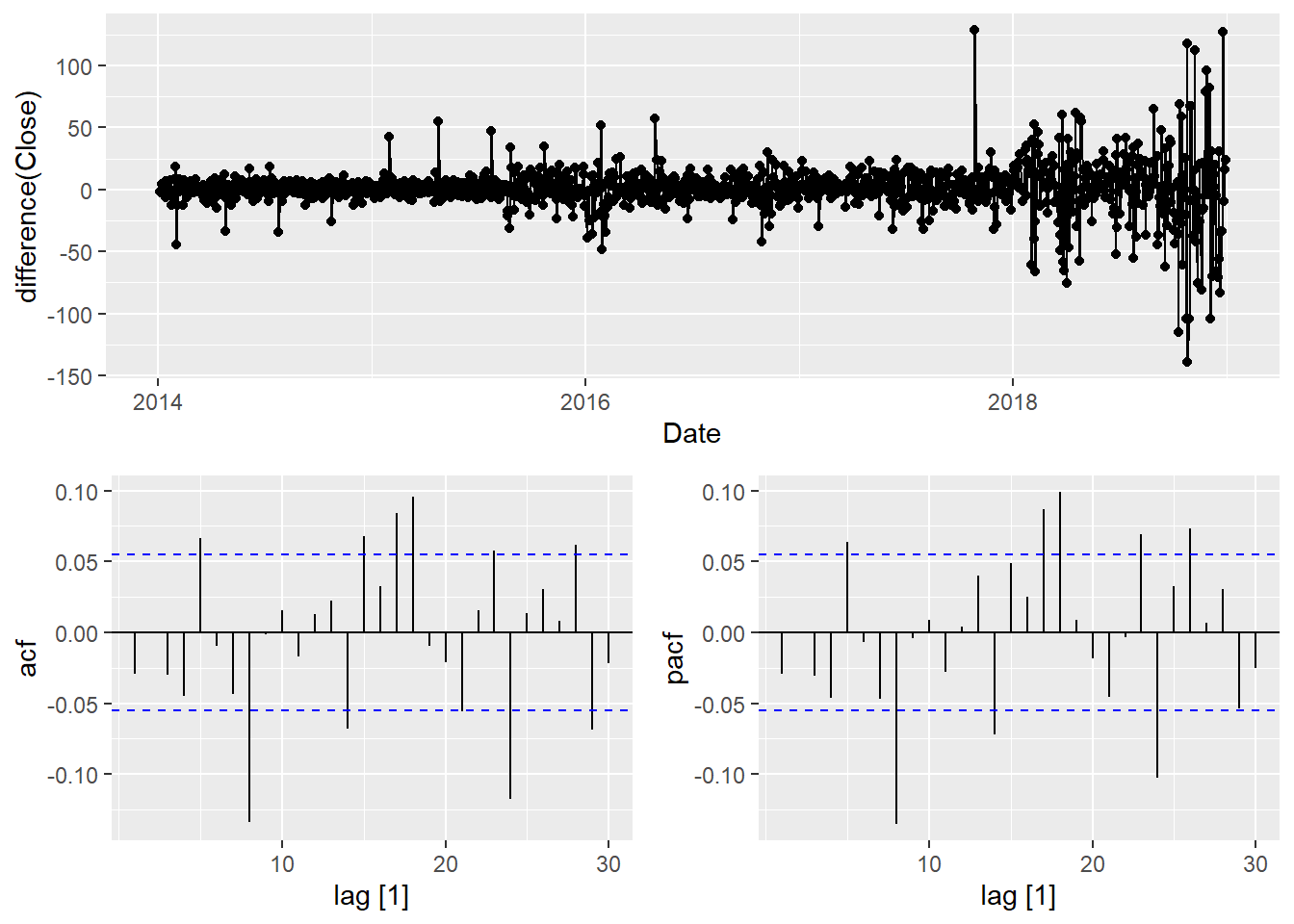9.3) For the following series, find an appropriate Box-Cox transformation and order of differencing in order to obtain stationary data.

a. Turkish GDP from global_economy.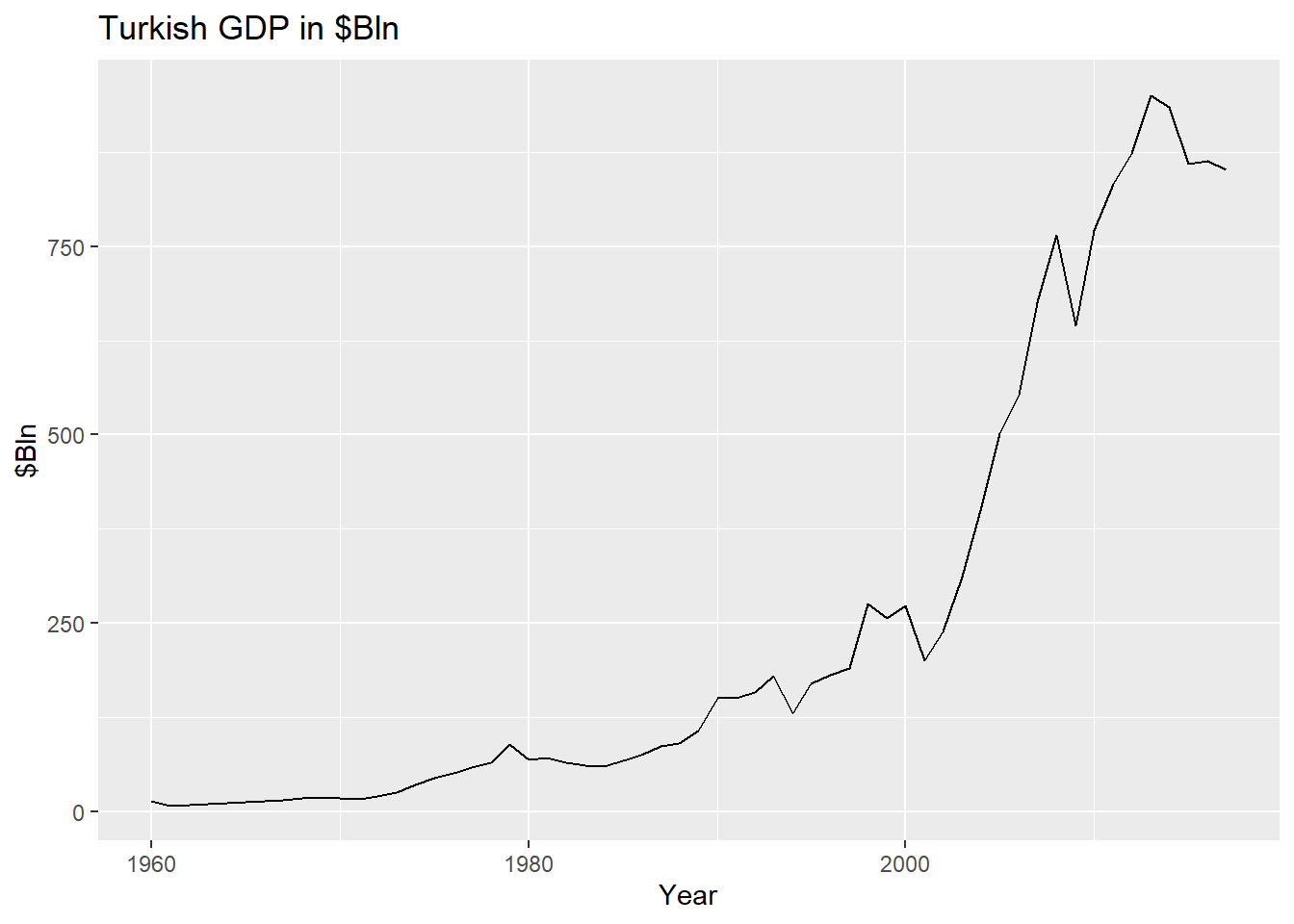The Turkish GDP shows am upward trend that gets strong after 2001. Let’s apply the Box Cox transformation.

##  0.1572187

The optimal lambda value for the Box Cox tranformation is 0.1572. We now calculate first differences of the transformed series.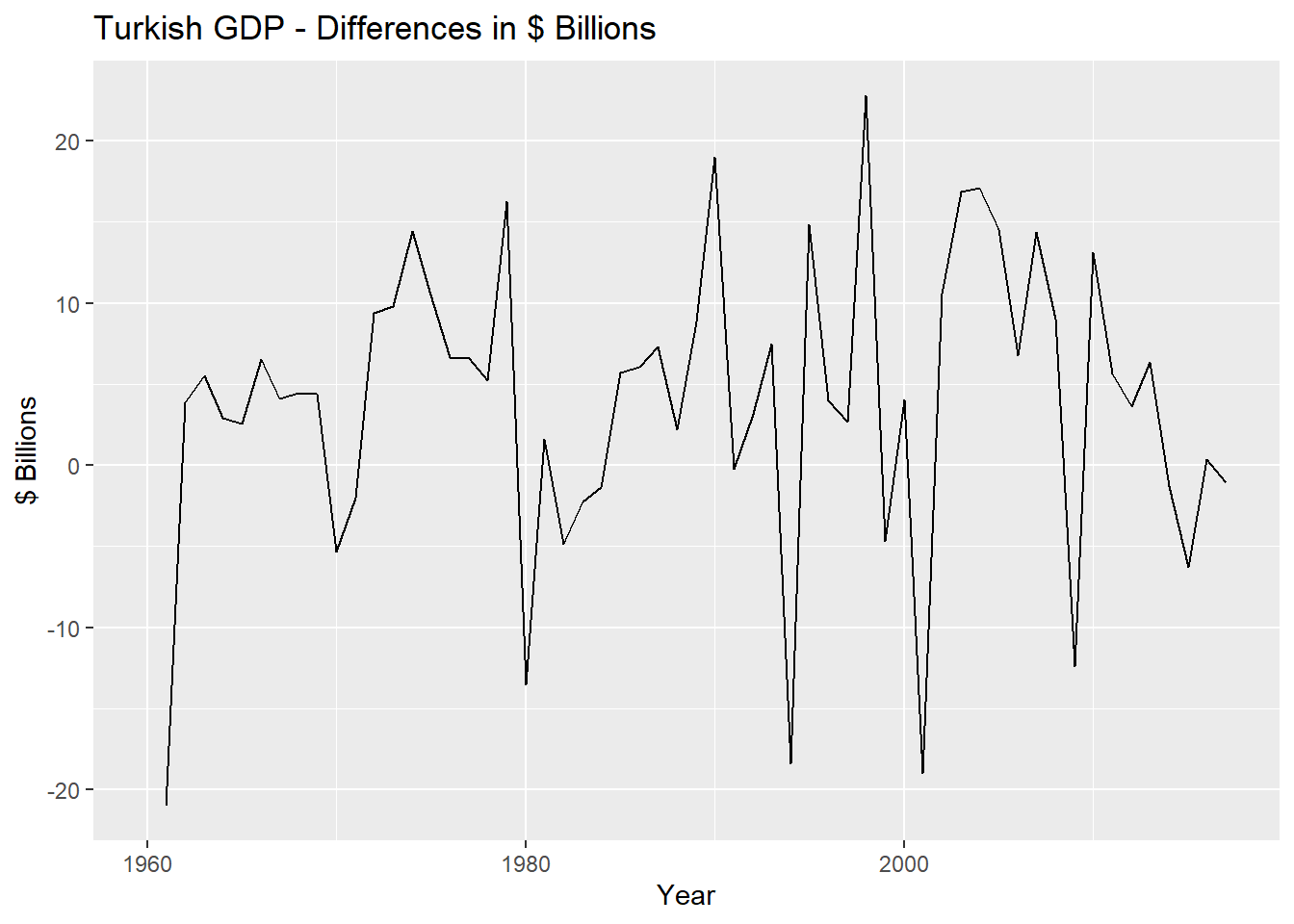We run the KPSS test for stationarity

## # A tibble: 1 x 3
##   Country kpss_stat kpss_pvalue
##   <fct>       <dbl>       <dbl>
## 1 Turkey     0.0889         0.1

Based on the test, we can conclude that the time series is now stationary.

b. Accommodation takings in the state of Tasmania from aus_accommodation.

## # A tsibble: 6 x 5 [1Q]
## # Key:       State 
##      Date State                        Takings Occupancy   CPI
##     <qtr> <chr>                          <dbl>     <dbl> <dbl>
## 1 1998 Q1 Australian Capital Territory    24.3        65  67
## 2 1998 Q2 Australian Capital Territory    22.3        59  67.4
## 3 1998 Q3 Australian Capital Territory    22.5        58  67.5
## 4 1998 Q4 Australian Capital Territory    24.4        59  67.8
## 5 1999 Q1 Australian Capital Territory    23.7        58  67.8
## 6 1999 Q2 Australian Capital Territory    25.4        61  68.1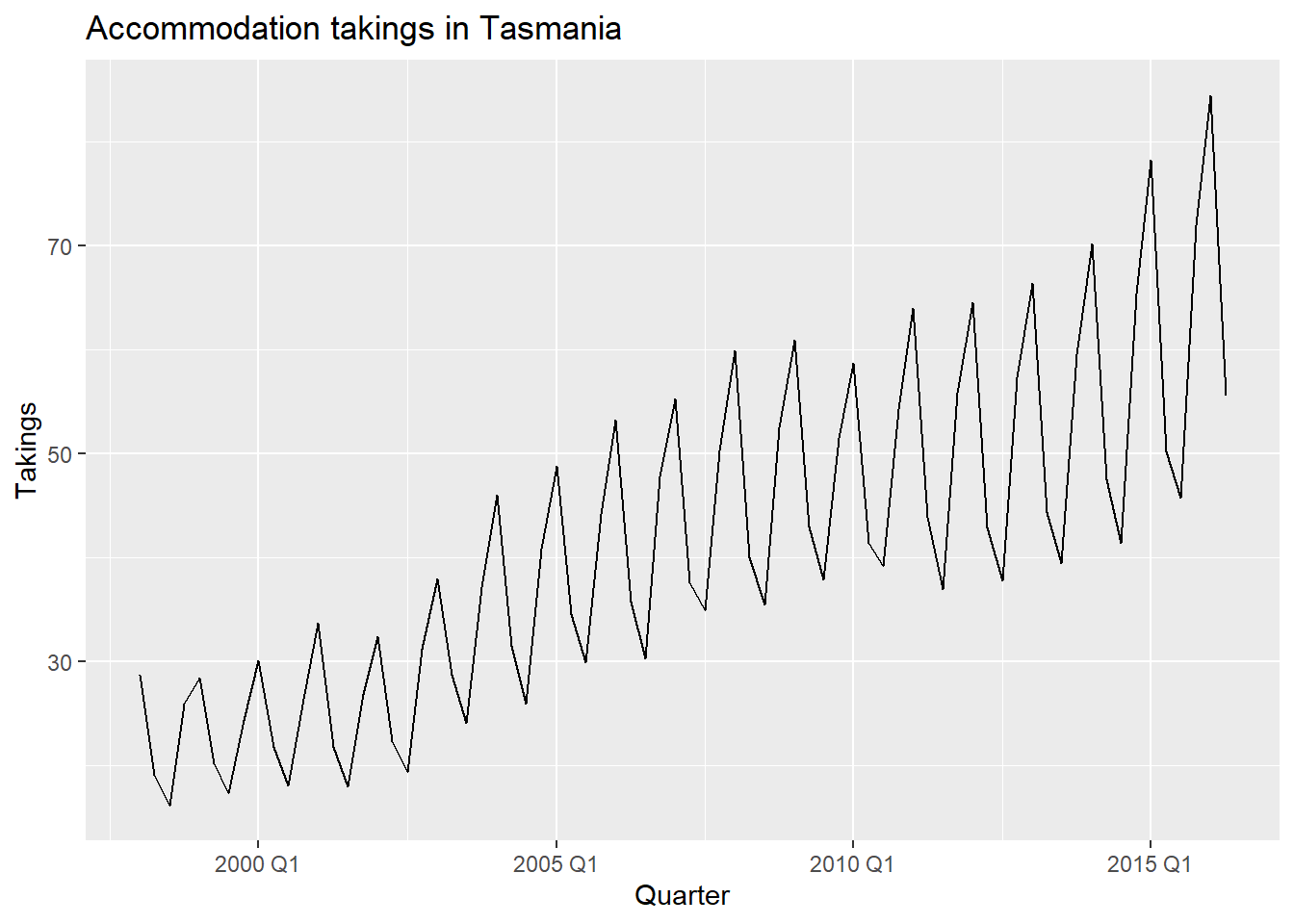The above plot shows an upward trend, strong seasonality as well as increasing variance. We apply the Box Cox transformation.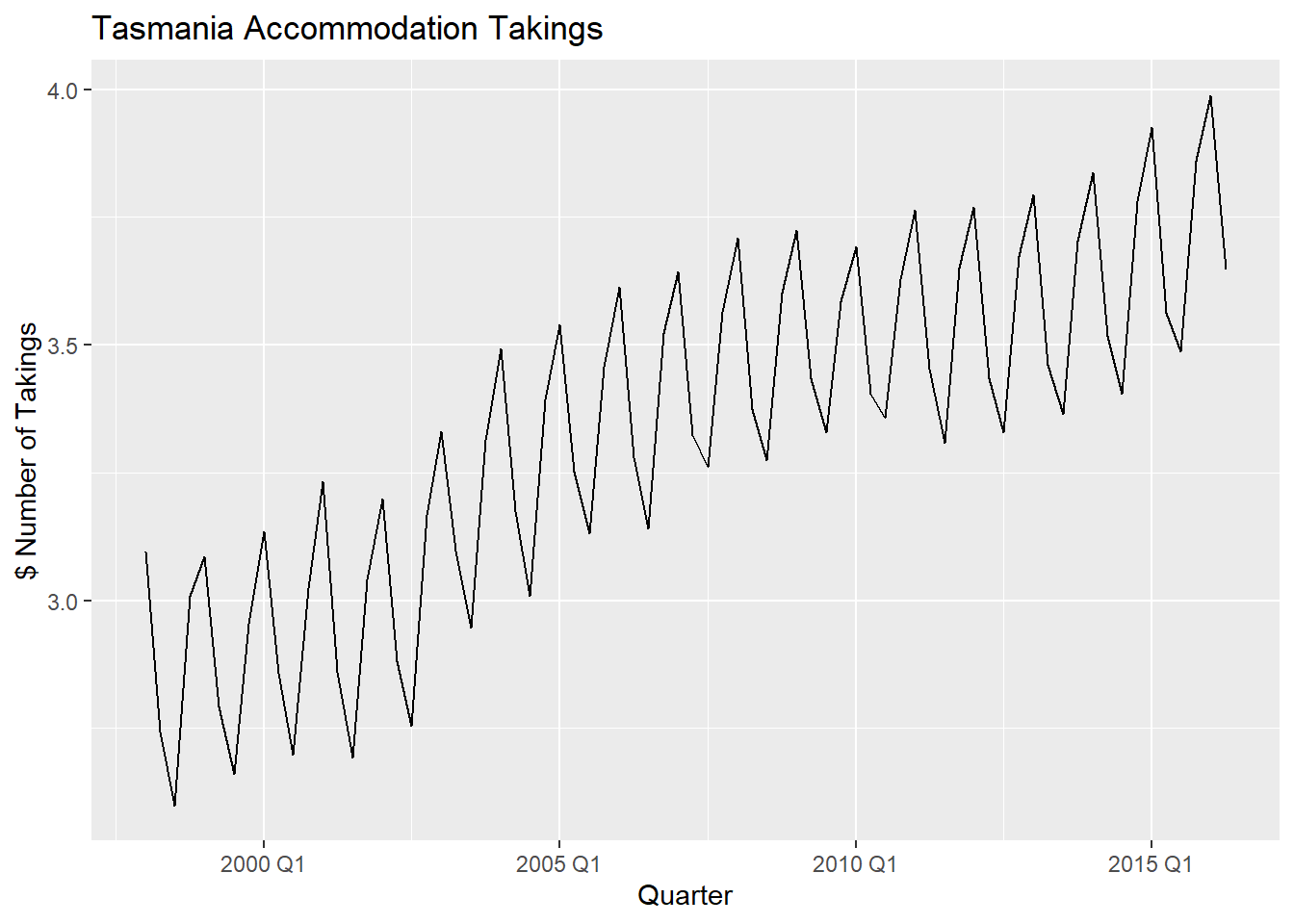This seems to have stabilized the variance, but there is still an upward trend.

##  -0.04884781

The optimal lambda avalue is -0.0488.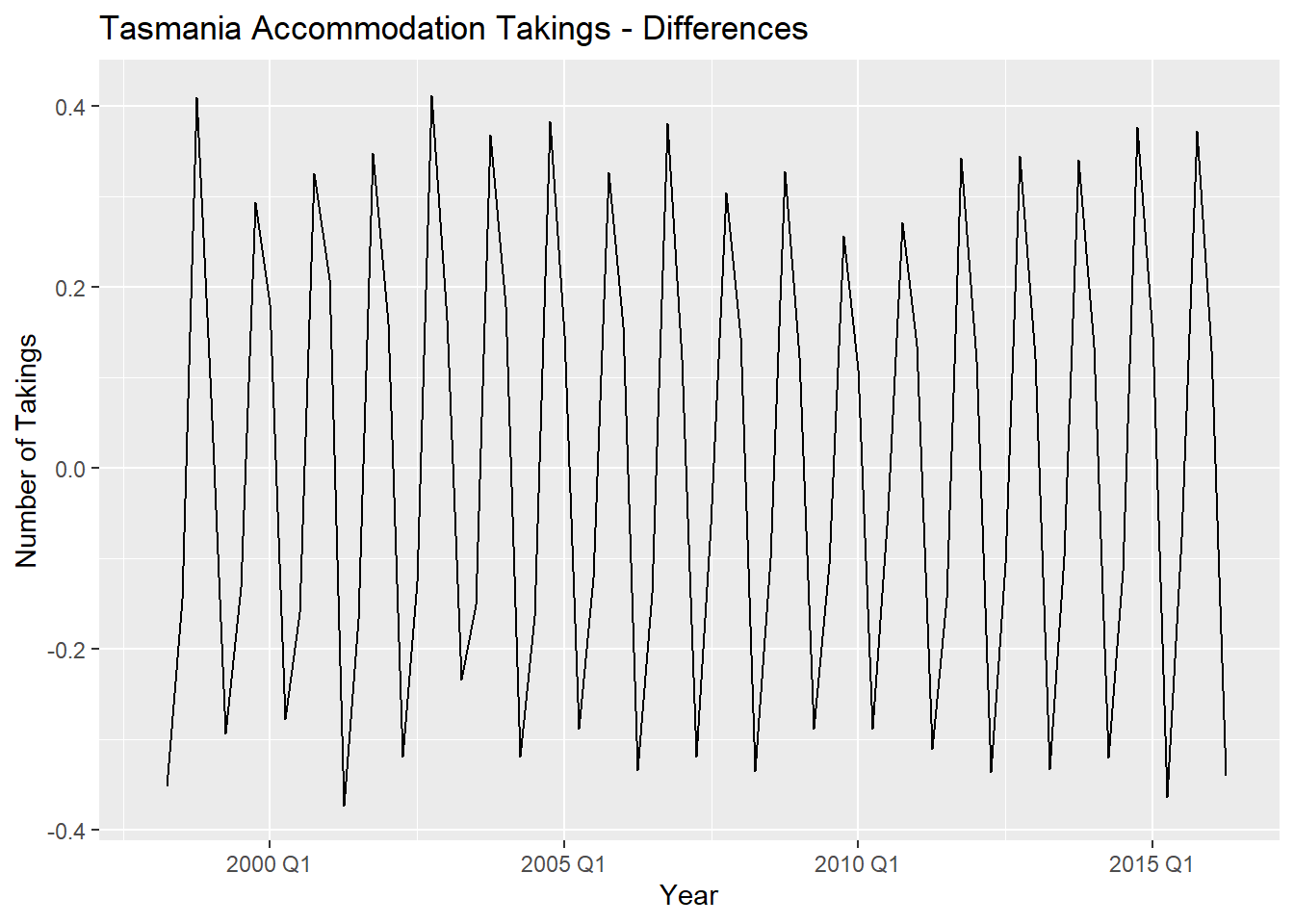After taking first differences of the transformed data, the upward trend has been removed. We run the KPSS test for stationarity

## # A tibble: 1 x 3
##   State    kpss_stat kpss_pvalue
##   <chr>        <dbl>       <dbl>
## 1 Tasmania     0.256         0.1

Based on the test, we can conclude that the time series is now stationary.

c. Monthly sales from souvenirs.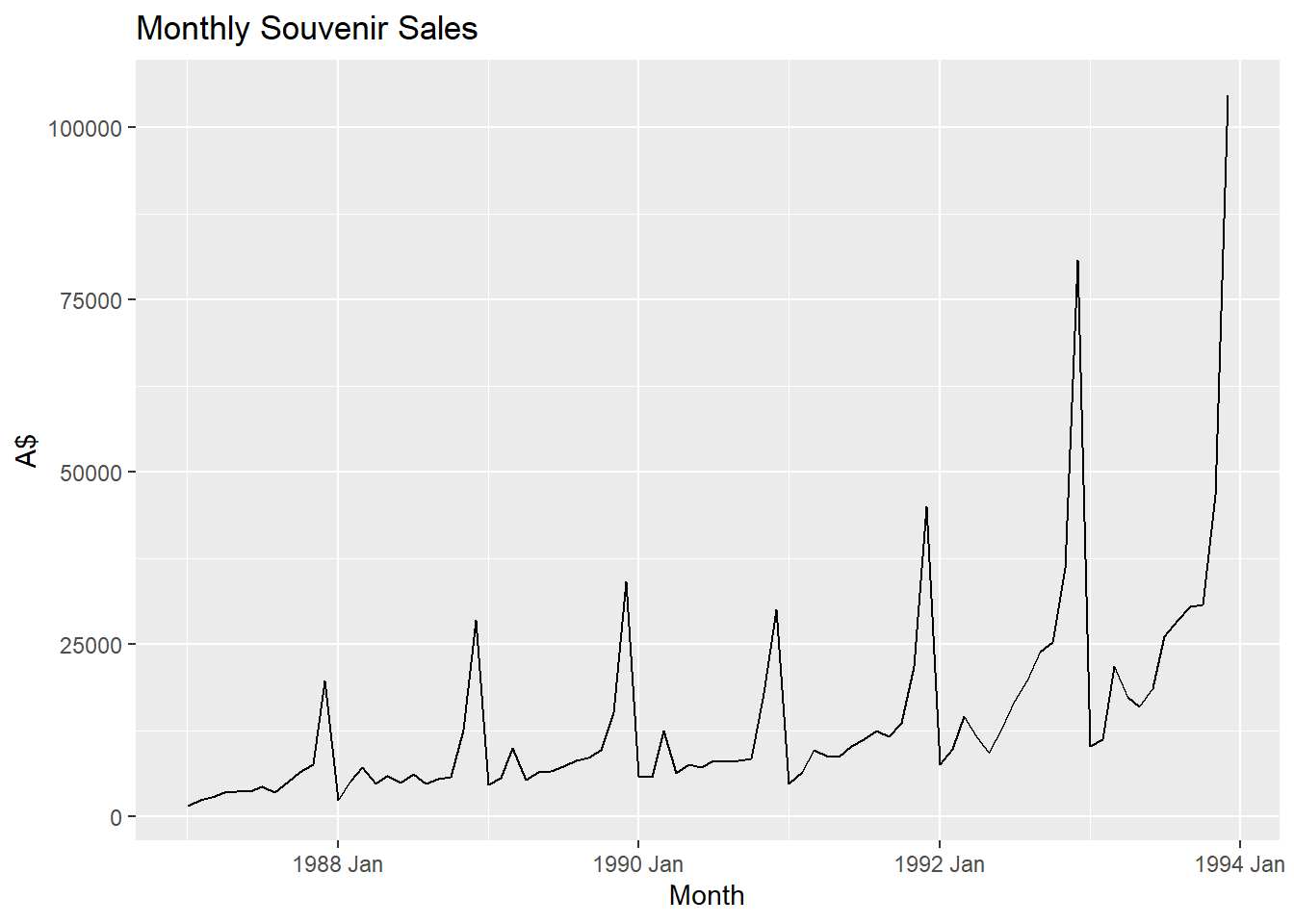The souvenirs data shows an upward trend, strong seasonality and an increasing variance. Let’s apply the Box Cox transformation.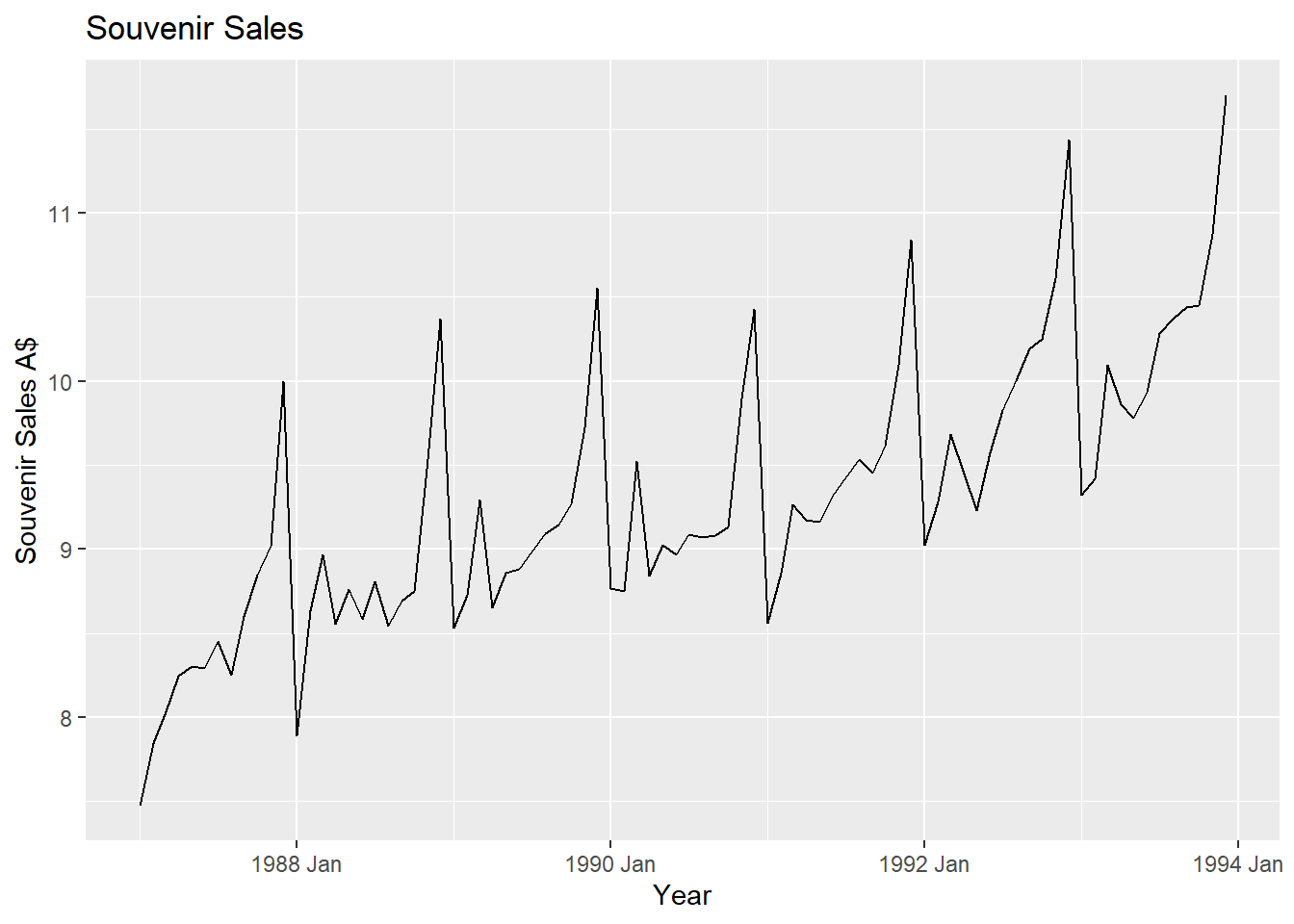This transformation seems to have stabilized the variance.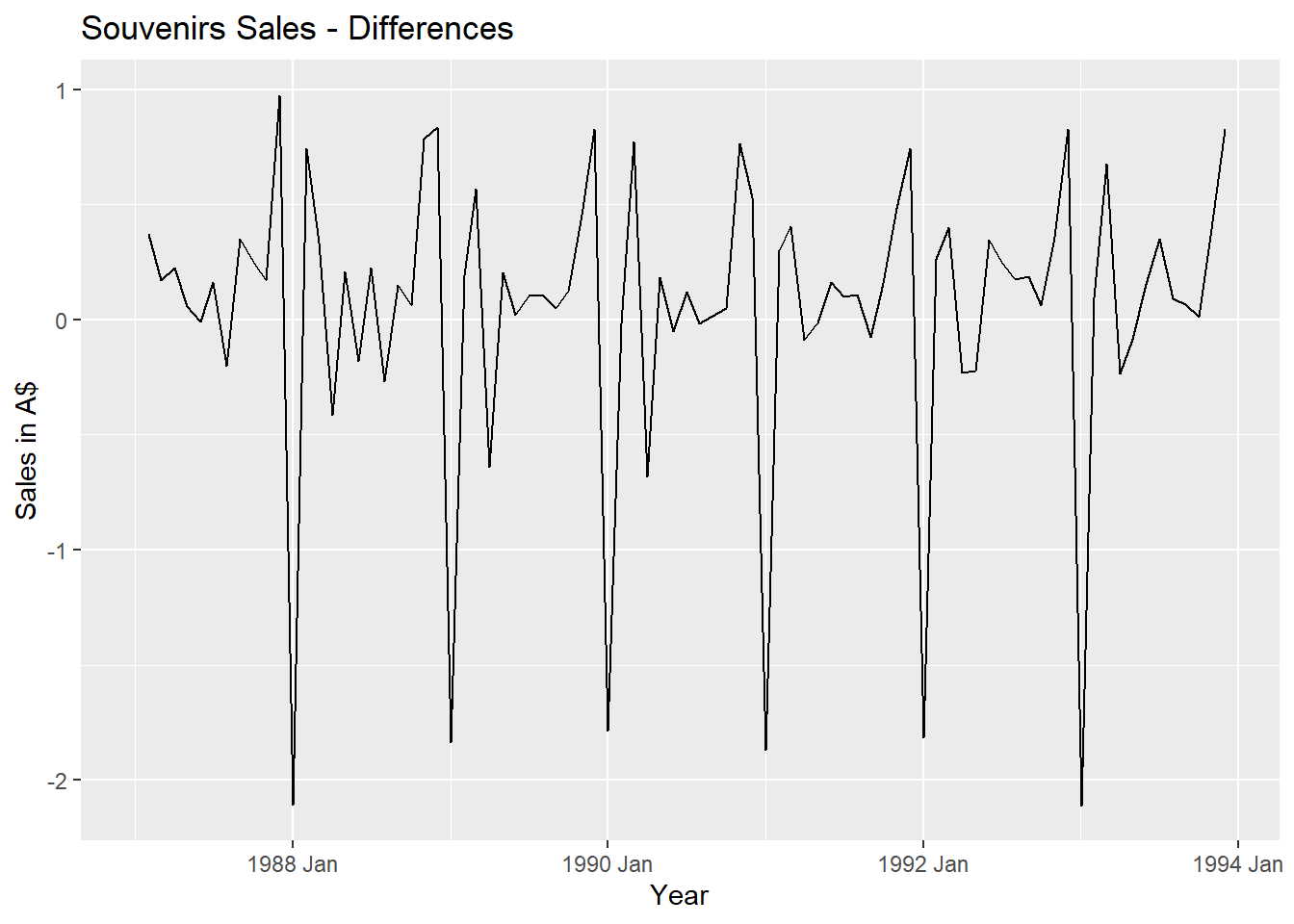Taking first differences of the transformed data has removed the upward trend.

We run the KPSS test for stationarity

## # A tibble: 1 x 2
##   kpss_stat kpss_pvalue
##       <dbl>       <dbl>
## 1    0.0631         0.1

Based on the test, we can conclude that the time series is now stationary.

##9.5) For your retail data (from Exercise 8 in Section 2.10), find the appropriate order of differencing (after transformation if necessary) to obtain stationary data.